Question of The Day11-07-2022

When a trader sold an article at a discount of 20% on the marked price. He offers an article for free on buying 9 articles. In the whole transaction, he gets a profit of 26 %. Find the percentage increase in marked price from the cost price.

Correct Answer : b ) 75%

Explanation :

Let the cost price and marked price of the article be Rs. 100 and Rs. x respectively.

According to the question, trader gives 20 % discount on the marked price.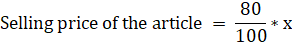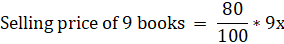Cost price of 10 books = 100 * 10

Since, trader earn 26% profit in whole transaction.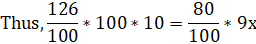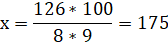Marked price of the article = Rs. 175

We assumed the cost price of article as Rs. 100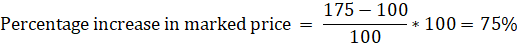Therefore, 75% is increased in marked price from cost price.

Hence, (b) is the correct answer.0